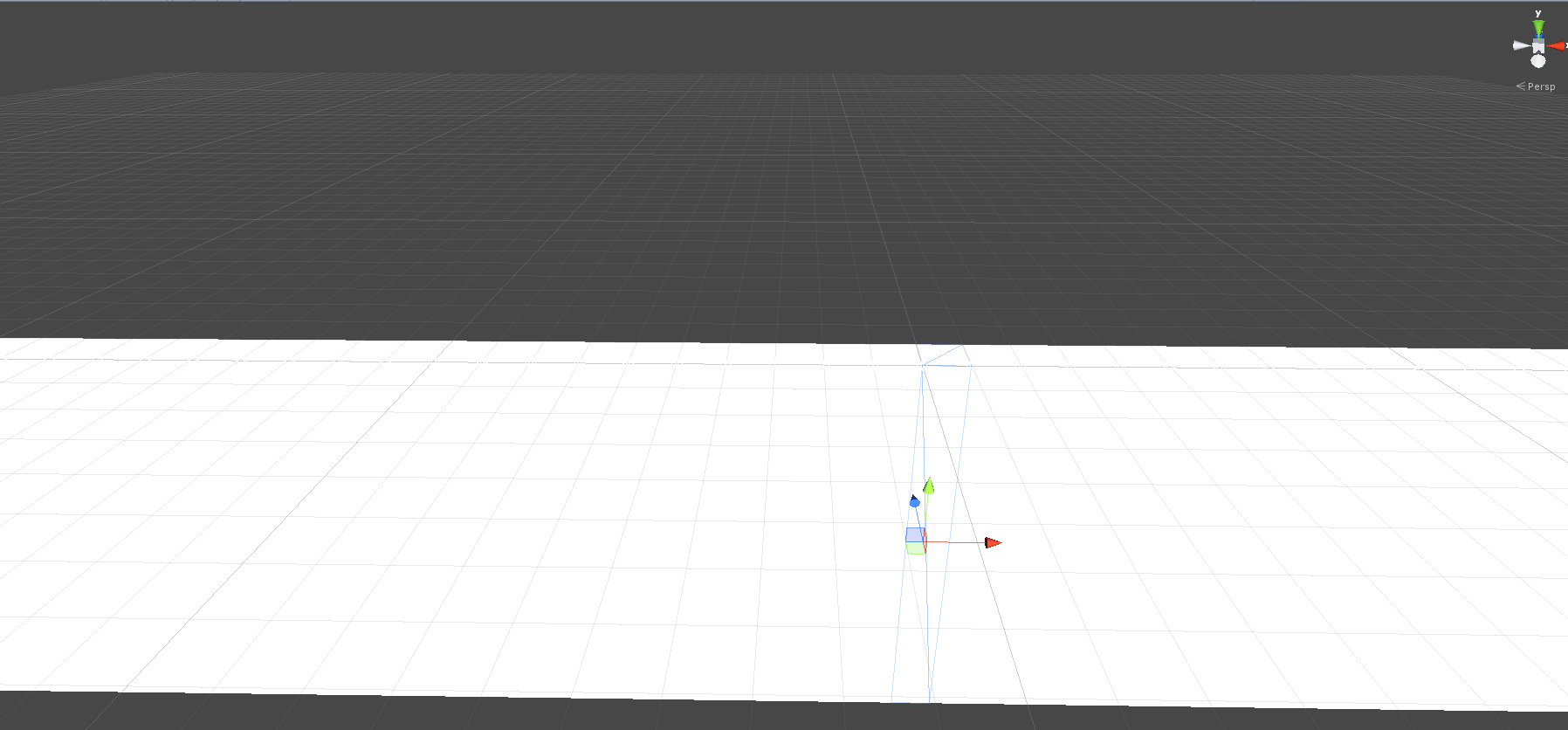I just managed to have a 2d water line in Unity 4.3.

I’d like to share a good working base with you, it’s not a ready to use solution but it can be a good start for a more complex water line.

It’s not that hard to make, but it lacks of buoyancy, droplets, reflection… like I said, it’s a start.

## The idea

The line is a list of dots connected by springs.

Then we create a simple mesh (few triangles) between each pair of dots.

Finally, we update the mesh vertices at each update using the new dots positions.

## The result

Here’s the result with the `Sprite-default` material:You can probably apply some shader on it.

## The script

See on Gist

``````// Author: Damien Mayance (http://dmayance.com)
// 2013 - Pixelnest Studio (http://pixelnest.io)
//
// This script simulates a simple 2d water line behavior, like you see in many 2d games.
// See http://dmayance.com/water-line-2d-unity/ for further explanations.
//
// Usage:
// - Attach it to an object
// - Then fill the "Material" parameter
// - Start the game (here it is only visible at runtime)
//
// The parameters can be modified during runtime, but for EDITOR only.
// The width and height should stay fixed during the game otherwise.
using UnityEngine;
using System.Collections;

public struct WaterLinePart
{
public float height;
public float velocity;
public GameObject gameObject;
public Mesh mesh;
public Vector2 boundsMin;
public Vector2 boundsMax;
}

public class WaterLine : MonoBehaviour
{
public float velocityDamping = 0.999999f; // Proportional velocity damping, must be less than or equal to 1.
public float timeScale = 25f;

public int Width = 50;
public float Height = 10f;
public Material material;
public Color color = Color.blue;

private WaterLinePart[] parts;

private int size;
private float currentHeight;

#if UNITY_EDITOR
private bool cleanRequested;
#endif

void Start()
{

#if UNITY_EDITOR
// Remove what we see from the editor
Clear();
#endif

Initialize();
}

private void Initialize()
{
size = Width;
currentHeight = Height;

material.color = color;

parts = new WaterLinePart[size];

// we'll use spheres to represent each vertex for demonstration purposes
for (int i = 0; i < size; i++)
{
// Create a game object
GameObject go = new GameObject("WavePart");
go.transform.parent = this.transform;
go.transform.localPosition = new Vector3(i - (size / 2), 0, 0);

parts[i].gameObject = go;
}

// Create the meshes
for (int i = 0; i < size; i++)
{
GameObject go = parts[i].gameObject;

// Except for the last point
if (i < size - 1)
{
Mesh mesh = new Mesh();
mesh.MarkDynamic();
parts[i].mesh = mesh;

// Define vertices for the mesh (the points of the model)
UpdateMeshVertices(i);

// Define triangles and normals
InitializeTrianglesAndNormalsForMesh(i);

go.GetComponent<MeshFilter>().mesh = mesh;
go.GetComponent<MeshRenderer>().material = material;
}
}

// Small wave
Splash(size / 2, 10);
}

#if UNITY_EDITOR
/// <summary>
/// SUPER VIOLENT METHOD FOR EDITOR MODE
/// </summary>
private void Clear()
{
for (int i = 0; i < size; i++)
{
DestroyImmediate(parts[i].mesh);
DestroyImmediate(parts[i].gameObject);
}

parts = null;
}
#endif

private void UpdateMeshVertices(int i)
{
Mesh mesh = parts[i].mesh;
if (mesh == null) return;

Transform current = parts[i].gameObject.transform;

Transform next = current;
if (i < parts.Length - 1)
{
next = parts[i + 1].gameObject.transform;
}

Vector3 left = Vector3.zero;
Vector3 right = next.localPosition - current.localPosition;

// Get all parts of the mesh (it's just 2 planes, one on top and one on the front face)
Vector3 topLeftFront = new Vector3(left.x, left.y, 0);
Vector3 topRightFront = new Vector3(right.x, right.y, 0);
Vector3 topLeftBack = new Vector3(left.x, left.y, 1);
Vector3 topRightBack = new Vector3(right.x, right.y, 1);
Vector3 bottomLeftFront = new Vector3(left.x, left.y + (0 - Height), 0);
Vector3 bottomRightFront = new Vector3(right.x, right.y + (0 - Height), 0);

mesh.vertices = new Vector3[] { topLeftFront, topRightFront, topLeftBack, topRightBack, bottomLeftFront, bottomRightFront };

parts[i].boundsMin = topLeftFront + current.position;
parts[i].boundsMax = bottomRightFront + current.position;
}

private void InitializeTrianglesAndNormalsForMesh(int i)
{
Mesh mesh = parts[i].mesh;
if (mesh == null) return;

// Normals
var uvs = new Vector2[mesh.vertices.Length];
for (int i2 = 0; i2 < uvs.Length; i2++)
{
uvs[i2] = new Vector2(mesh.vertices[i2].x, mesh.vertices[i2].z);
}
mesh.uv = uvs;

// Triangles
mesh.triangles = new int[] { 5, 4, 0, 0, 1, 5, 0, 2, 3, 3, 1, 0 };

mesh.RecalculateNormals();
}

void Update()
{
#if UNITY_EDITOR
// Size has been updated?
if (Width != size || Height != currentHeight)
{
cleanRequested = true;
}

// Recalculate everything!
// This should be for the editor only!
if (cleanRequested)
{
cleanRequested = false;
Debug.Log("Reinitializing water. Make sure we are in editor mode!");
Clear();
Initialize();
}

color = material.color;
#endif

// Water tension is simulated by a simple linear convolution over the height field.
for (int i = 1; i < size - 1; i++)
{
#if UNITY_EDITOR
// Objects deleted from editor
if (parts[i].gameObject == null)
{
cleanRequested = true;
return;
}
#endif
int j = i - 1;
int k = i + 1;
parts[i].height = (parts[i].gameObject.transform.localPosition.y + parts[j].gameObject.transform.localPosition.y + parts[k].gameObject.transform.localPosition.y) / 3.0f;
}

// Velocity and height are updated...
for (int i = 0; i < size; i++)
{
// update velocity and height
parts[i].velocity = (parts[i].velocity + (parts[i].height - parts[i].gameObject.transform.localPosition.y)) * velocityDamping;

float timeFactor = Time.deltaTime * timeScale;
if (timeFactor > 1f) timeFactor = 1f;

parts[i].height += parts[i].velocity * timeFactor;

// Update the dot position
Vector3 newPosition = new Vector3(
parts[i].gameObject.transform.localPosition.x,
parts[i].height,
parts[i].gameObject.transform.localPosition.z);
parts[i].gameObject.transform.localPosition = newPosition;
}

// Update meshes
for (int i = 0; i < size; i++)
{
UpdateMeshVertices(i);
}
}

#region Interaction

/// <summary>
/// Make waves from a point
/// </summary>
/// <param name="location"></param>
/// <param name="force"></param>
public void Splash(Vector3 location, int force)
{
// Find the touched part
for (int i = 0; i < (size - 1); i++)
{
if (location.x >= parts[i].boundsMin.x
&& location.x < parts[i].boundsMax.x)
{
if (location.y <= parts[i].boundsMin.y
&& location.y > parts[i].boundsMax.y)
{
Splash(i, force);
}
}
}

}

private void Splash(int i, int heightModifier)
{
parts[i].gameObject.transform.localPosition = new Vector3(
parts[i].gameObject.transform.localPosition.x,
parts[i].gameObject.transform.localPosition.y + heightModifier,
parts[i].gameObject.transform.localPosition.z
);
}

#endregion
}
``````

Enjoy! ;)